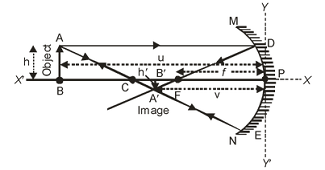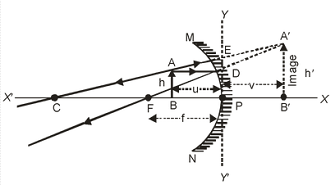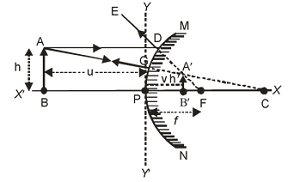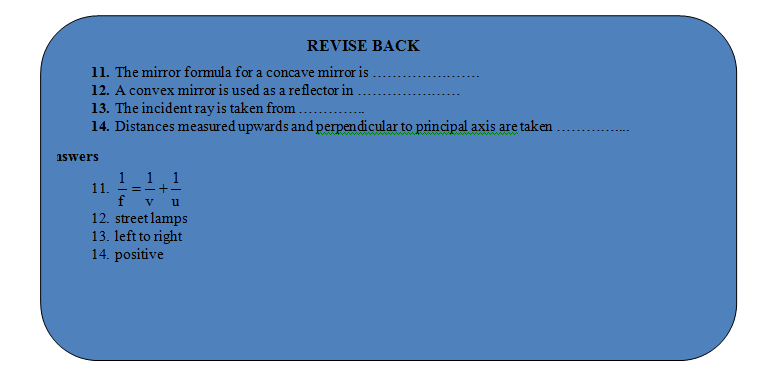# IMPORTANT CONCEPT

## Light of Class 9

### MPORTANT CONCEPT

On the basis of the New Cartesian Sign Convention discussed above, we can draw the following conclusions for the spherical mirrors (concave as well as convex mirrors).

## IN THE CASE OF CONCAVE MIRROR:

(i) For real and inverted image

 Focal length(f)= –ve Radius of curvature(R)= –ve Object distance (u)= –ve Object height(h)= +ve Image distance (v)= –ve Image height(h′)=–ve Magnification (m)= –ve(ii) For virtual and erect image

 focal length(f)= –ve Radius of curvature(R)= –ve Object distance(u)= –ve Object height(h)= +ve Image distance(v)= +ve Image height(h′)= +ve Magnification (m)= +ve### IN CASE OF CONVEX MIRROR

A convex mirror always forms a virtual and erect image.

 Therefore for virtual and erect image: Focal length (f)= +ve Radius of curvature (R)=+ve Object distance (u)=–ve Object height (h)= +ve Image distance (v)=+ve Image height (h′)=+ve Magnification (m)= +veIn the case of a convex mirror, for virtual and erect image, only object distance (u) is –ve and the remaining all distances are +ve.